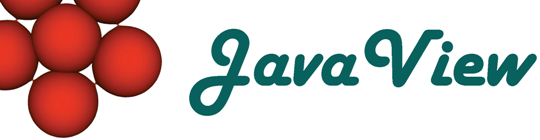Overview  Animation  Classic Surfaces  Parm Surfaces  Curves on Surfaces  Discrete Geodesics  ODE  Platonic Solids  Cycloid  Root Finder  Harmonic Maps  Rivara Bisection  Scalar Field  Weierstrass  Closed Polygon  Elastic Curve  Billiard in an Ellipse  LIC Visualization  Discrete VF  Hodge Splitting  Textured Surface  Surfaces of Rotation  Mean Curvature Flow  Pythagoraen Tree  Julia Sets

## Line Integral Convolution

Uses a geodesic Line Integral Convolution method to visualize vector fields. The image is created beginning with a white noise that is then convoluted along integral lines of the given vector field. That creates a visible correlation between image pixels that lie on the same integral line. The integral lines are calculated with an geodesic 4th or 1st order Runge-Kutta method (that's the difference between fast mode and non-fast mode).

Line Integral Convolution

In this example a potential creates the vector field. The centers of the potential are the vertices of a point set. Keep pressing key 'a' while clicking the left mouse button to add a vertex of type as specified at 'Vertex Type'. Click and drag single vertices to move them around.

To initiate the LIC image calculation press button 'Compute LIC Image'. There many parameters that control the behavior of the LIC method:

 Fast (Euler) Mode If this switch is activated then instead of a 4th order Runge-Kutta integration a 1st order (Euler-) method is used. Convolution Width This specifies the "normal" width of the convolution window in pixels that is modified with the velocity of the vector field at every point. Min. Convolution Width Minimum convolution window size in pixels. Noise Coarseness As said the coarseness of the noise: value of 1 is a "noise" that has a constant color, value 0 makes the noise uncorrelated (maximal chaotic). LIC Size The width in pixels of the LIC texture image (it has the same height). This is not a direct measurement of the pixels actually used for the visible element textures but indirect it is (in some cases nearly half of the pixels in the texture image are unused).

The other panel named 'Grid' allows to change the diameter and discretization of the domain.